[TOC]

# 1. Introduction# 2. Observations

ex. 3-SUM pb
given N distinct numbers, how many triples sum up to 0? (pb related to computatioal geogtry)

• brute force method:
```for(int i=0;i<N;i++)
for(int j=i+1;j<N;j++)
for(int k=j+1;k<N;k++)
{if(a[i]+a[j]+a[k]==0)
count++;
}
```
• mesuring running time:

stdlib.jar里面提供了一个`Stopwatch`类用于记录运行时间.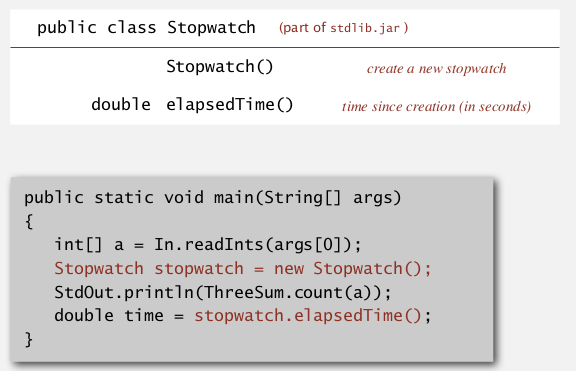• log-log plot

T(N) = running time for input of size N
log(N)-log(T(N)) plot:
often get a straight line — power law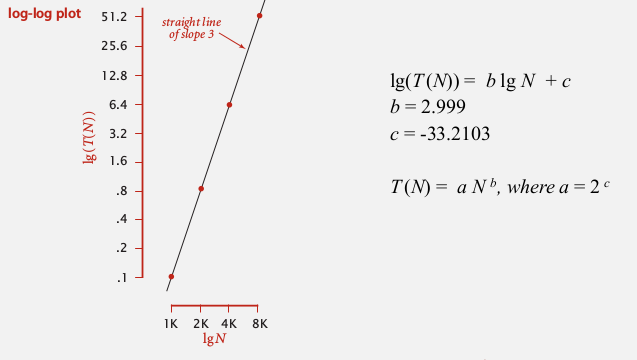• doubling ratio:

(for checking the power law relationship, checking the power order)
each time double the size of input, then take log of the time ratio of 2 runs: log( T(2N)/T(N) )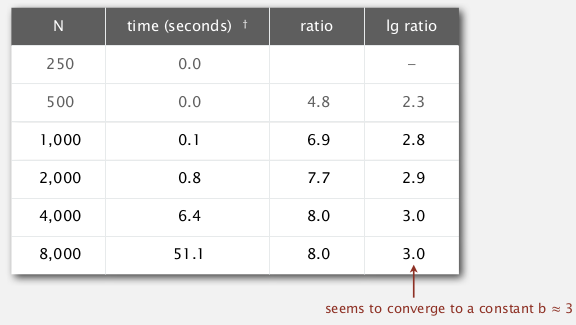# 3.Mathematical Models

• total running time: sum of cost*frequency of operations
• cost of some basic operations:

• array allocation: c*N (because all array entries have to be set to 0/false/null)
• string concatenation: c*N (proportional to the length of string !)
• simplification

crude analysis
ignore lower terms tilde notation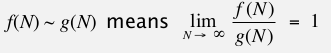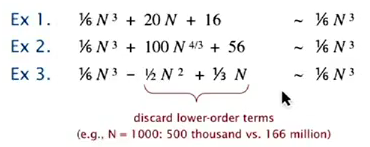• estimating discrete sum by relaxation

Replace the sum with an integral, and use calculus — 很机智...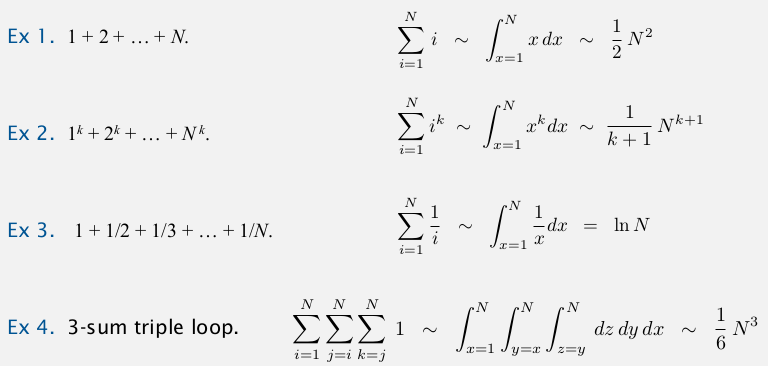# 4. Order of Growth Classification

(discard the leading coefficient when considering the growth order)

• only a small set of growth functions:

`1, logN, N, NlogN, N^2, N^3, 2^N`• exemples:

binary search ⇒ logN
divide and conquer ⇒ NlogN
exhaustive search ⇒ 2^N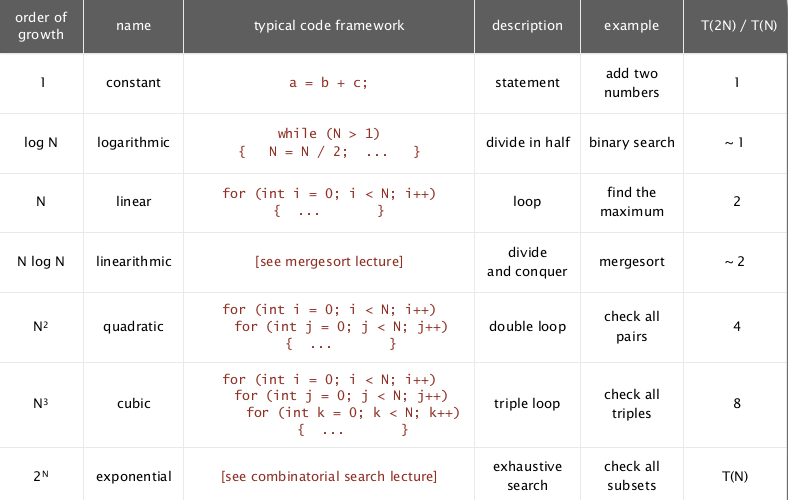practical performance: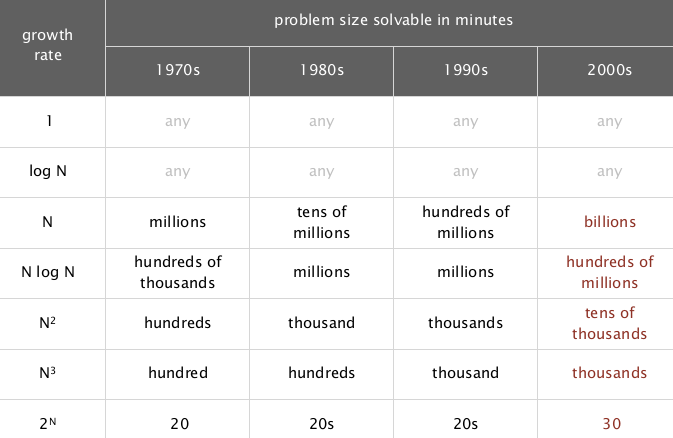• ex. binary search

public int binearch(int arr[], int key){//arr[] already sorted int lo=0,hi=arr.length; while(i<j){ int m = (lo+hi)/2; if(arr[m]==key) return m; else if(arr[m]<key) lo=m+1; else hi=m-1; } return -1; }

(→ Bug in Java's Arrays.binarySearch() discovered in 2006......)
→ invariant: if key in arr, arr[lo]<=key<=arr[hi]
proposition. binary search uses at most logN+1 compares to search a sorted array of size N. pf. denote T(N) := nb of compares for array with size <=N
→ T(1)=1
→ recurrence relation: T(N)<=T(N/2)+1
⇒ T(N)=logN

• a faster 3-SUM

→ first sort the array (~NlogN)
→ for any pair a[i] and a[j], do binary search for -(a[i]+a[j]) ~(N2LogN)
⇒ reduce from N3 to N2logN ! (for 8k numbers, running time goes from 51s to 0.96s)

# 5. Theory of Algorithms

• types of analysis

-best case -worst case -average case(random input, "expected cost")

• notations

big Theta/big O/big Omega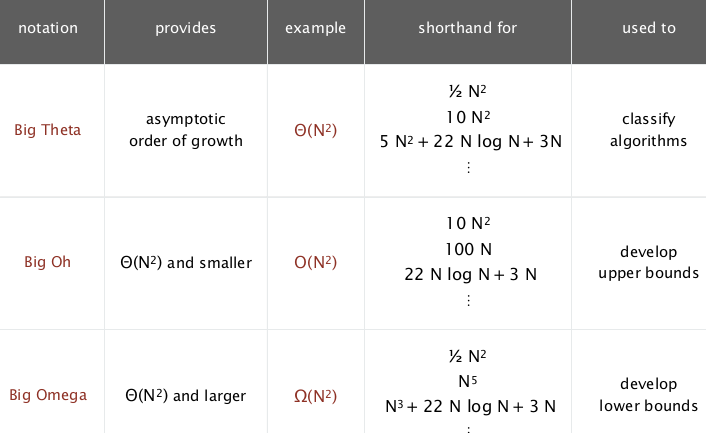- big O: upper bound → * once a specific algo is found, find an upper bound
- big Omega:
lower bound → proove that no algo can do better
- big Theta: symptotic growth (same order, optimal algo) → lower and upper bound
match*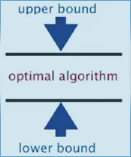⇒ in this course: use tilde notation: contain leading constants for highest order term

# 6. Memory

KB: 2^10 bytes
MB: 2^20 bytes (1 million) GB: 2^30 bytes (1 billion) 64-bit machines: 8 byte pointers

• typical memory usage:

for primary types: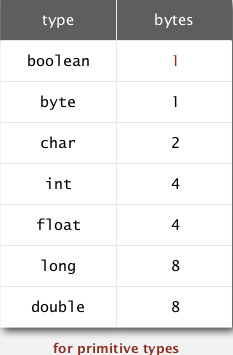for arrays (with array overhead=24bytes) :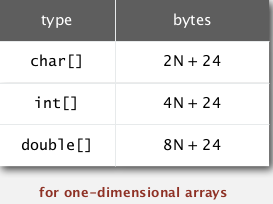Obj overhead: 16 bytes (obj的大小=16+obj内部filed的大小)
references: 8 bytes (ex. inner class has a ref to encolsing class)
padding: each obj uses a multiply of 8 bytes (obj大小=8 bytes的整数倍)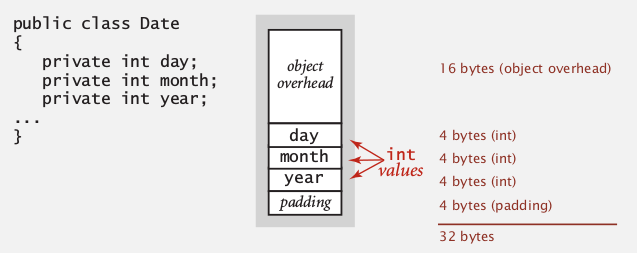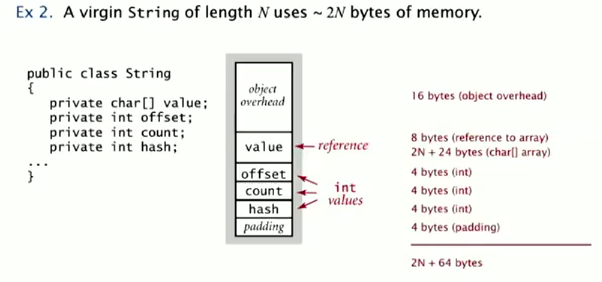#### Part 2 of series «Algorithms Princeton MOOC I»：

comments powered by Disqus